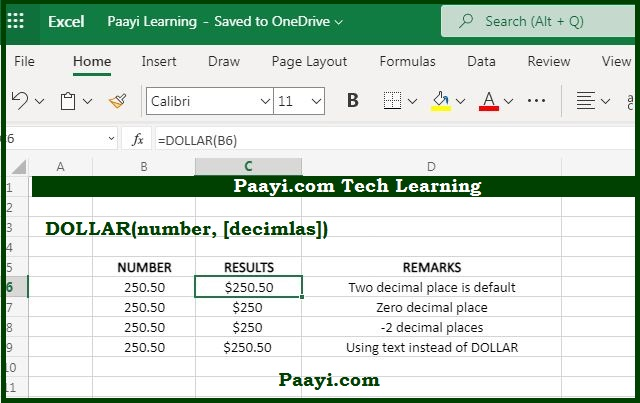# Learn How to Use Microsoft Excel DOLLAR Function

Written by | 0 Comments | 820 Views

In this article, you will learn how to use the Microsoft Excel DOLLAR function and its prime function in Microsoft Excel. You will also get to know the Microsoft Excel DOLLAR function return value and syntax with the help of some examples.

## Microsoft Excel Dollar Function

The primary function of the Microsoft Excel DOLLAR function is to convert numbers as text and in currency format. With the help of the DOLLAR function, you can convert the number into text using a currency format. You can achieve this by using the TEXT function also, but it is more versatile than the DOLLAR function.

### DOLLAR Function Return Value

It will return a number as a text in a currency format.

DOLLAR Function Syntax

= DOLLAR (number, decimals)

Where the arguments:

• number: It is the number you want to convert.
• decimals: It is the number of digits to the right of the decimal point; the default is 2.

## How to Use Microsoft Excel DOLLAR Function?As we know, the DOLLAR function is used to convert the number as a text using the currency number format. Here is the example:

• \$#,##0.00_);(\$#,##0.00).

The default for decimal is 2; in case the number is negative, the number will be rounded off to the left of the decimal point. The name of the DOLLAR function and the currency symbol used is based on your computer language settings. But you can achieve this through the TEXT function, which is the more flexible option.